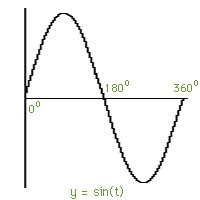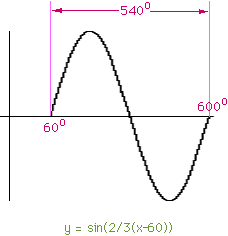Subject: graphs of other sine & cosine function Given; amplitute:1 period: 540 Phase shift: 60 degree,right I am ask to right the equation: sin 2/3 (value -60degree) When I am asked to graph the equation, the period is mixing me up. If you get this message before Chrism, Merry christmas and the best for the new year. if not best wish anyway. pierre Hi Pierre, To do this you need to keep in mind the graph of y = sin(t) for one period of the sine function, t = 0o to t = 360o.To graph y = sin(2/3(x-60o)) you can either use the given information: amplitute:1 period: 540 Phase shift: 60 degree,right or the expression y = sin(2/3(x-60o)). If you start with the information amplitute:1 period: 540 Phase shift: 60 degree,right then the phase shift information tells you to start the sine graph at +60o. The period being 540o means that one period of the sine function takes 540o. Thus this period is completed at 60o + 540o = 600o.If you have the expression y = sin(2/3(x-60o)) and not the information about period and phase shift then you can plot the graph as follows. Since you know the shape of y = sin(t) for t = 0o to t = 360o solve 2/3(x-60o) = 0 to get x = 60o and 2/3(x-60o) = 360o to get x = 600o. Thus the phase shift is 60o and the graph goes through one cycle from 60o to 600o. Hence its period is 600o - 60o = 540o. Cheers, Harley

Go to Math Central